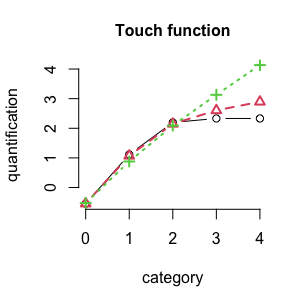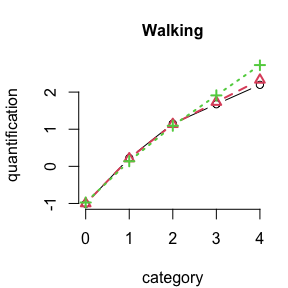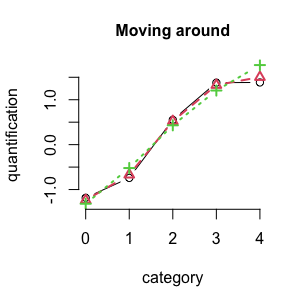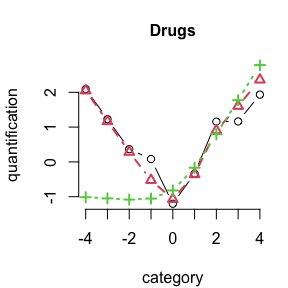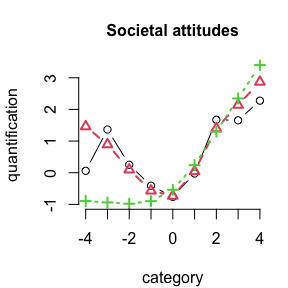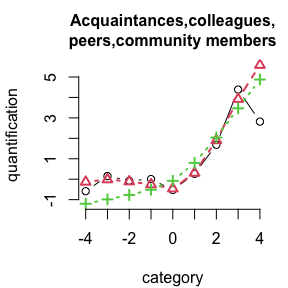# Introduction to ordPens: ordPCA

## Primary functions

• ordPCA
• ordFusion
• ordSelect
• ordSmooth
• ordAOV
• ordGene

The ordPens R package offers selection, and/or smoothing/fusing of ordinally scaled independent variables using a group lasso or generalized ridge penalty. In addition, nonlinear principal components analysis for ordinal variables is provided, using a second-order difference penalty.

For Nonlinear PCA, performance evaluation and selection of an optimal penalty parameter, use ordPCA(). Smoothing and selection of ordinal predictors is done by the function ordSelect(); smoothing only, by ordSmooth(); fusion and selection of ordinal predictors by ordFusion(). For ANOVA with ordinal factors, use ordAOV(). To test for genes that are differentially expressed between ordinal levels, use ordGene(). See also vignette("ordPens", package = "ordPens").

library(ordPens)

## Penalized nonlinear Principal Components Analysis

### EHD data example

ordPens offers, inter alia, nonlinear principal components analysis for ordinal variables. To explore the tools available for ordinal PCA, we’ll use some publicly available data sets. The ehd data from R package psy (Falissard, 2009) contains 269 observations of 20 ordinally scaled variables forming a polydimensional rating scale of depressive mood (Jouvent et al., 1988) and is documented in ?ehd.

To ensure adequate coding, we need to add +1 to the observed values.

library(psy)
data(ehd)
H <- ehd + 1
#>   e1 e2 e3 e4 e5 e6 e7 e8 e9 e10 e11 e12 e13 e14 e15 e16 e17 e18 e19 e20
#> 1  1  3  1  1  1  1  1  3  1   3   1   3   1   1   1   1   1   4   1   1
#> 2  3  3  3  1  1  1  4  1  4   1   5   1   5   5   1   1   1   1   1   1
#> 3  4  1  4  1  1  1  1  1  1   2   4   4   4   1   4   1   1   3   4   1
#> 4  4  3  3  1  1  1  3  1  2   3   4   3   4   1   4   1   3   4   4   4
#> 5  4  1  1  3  1  3  3  3  3   3   5   3   5   1   5   1   1   4   4   4
#> 6  4  1  1  4  4  3  1  1  1   1   4   4   4   1   4   1   1   3   4   4

The wrapper function ordPCA() handles both matrices or data frames of integers 1,2,…, giving the observed levels of the ordinal variables, as its first argument. The columns are by no means limited to have the same number of levels and can be specified by the argument Ks. Basically the algorithm converts the ordinal data to interval scale by finding optimal scores (known as optimal scoring/scaling in the context of nonlinear PCA, cf. Linting et al., 2007). Optimization, i.e. finding optimal quantifications/scores and principal components with corresponding loadings, is done via alternating least squares.

In order to take into account the ordinal character of the data, penalized ALS is applied where the amount of penalty can be controlled by the smoothing parameter lambda $$\in \mathbb{R}_0^{+}$$ using a second-order difference penalty. In addition, the function provides the option of both non-monotone effects and incorporating constraints enforcing monotonicity.

#### One dimensional estimation

Let’s start with the most basic example with univariate penalty, i.e. lambda being a scalar. We extract 2 principal components, specified by the argument p.

ehd_pca1 <- ordPCA(H, p = 2, lambda = 0.5, maxit = 100, crit = 1e-7,
qstart = NULL, Ks = apply(H, 2, max), constr = rep(TRUE, ncol(H)),
CV = FALSE, k = 5, CVfit = FALSE)

Extract the typical PCA summary (in alignment with base::prcomp()):

summary(ehd_pca1$pca) #> Importance of components: #> PC1 PC2 PC3 PC4 PC5 PC6 PC7 #> Standard deviation 2.3069 2.0727 1.28589 1.20784 1.06173 0.94744 0.83458 #> Proportion of Variance 0.2661 0.2148 0.08268 0.07294 0.05636 0.04488 0.03483 #> Cumulative Proportion 0.2661 0.4809 0.56356 0.63650 0.69286 0.73775 0.77257 #> PC8 PC9 PC10 PC11 PC12 PC13 PC14 #> Standard deviation 0.82072 0.73101 0.69502 0.65938 0.64164 0.62167 0.56776 #> Proportion of Variance 0.03368 0.02672 0.02415 0.02174 0.02058 0.01932 0.01612 #> Cumulative Proportion 0.80625 0.83297 0.85712 0.87886 0.89945 0.91877 0.93489 #> PC15 PC16 PC17 PC18 PC19 PC20 #> Standard deviation 0.54448 0.53235 0.47896 0.43652 0.41445 0.36142 #> Proportion of Variance 0.01482 0.01417 0.01147 0.00953 0.00859 0.00653 #> Cumulative Proportion 0.94971 0.96388 0.97535 0.98488 0.99347 1.00000 Estimated quantifications: ehd_pca1$qs
#> []
#>  -1.7679527 -0.8527782 -0.1482651  0.5575978  1.3476850
#>
#> []
#>  -0.8834568 -0.3020656  0.3328627  1.2297725  2.1423874
#>
#> []
#>  -0.5567114  0.8934859  1.5855562  2.0322602  2.4495844
#>
#> []
#>  -0.970451100 -0.427684089 -0.005904824  0.990089978  1.872762291
#>
#> []
#>  -0.7290737  0.1550859  0.7037962  1.5083075  2.5459961
#>
#> []
#>  -0.5801315  0.6767755  1.3387355  2.1641391  3.3464758
#>
#> []
#>  -0.81932720 -0.08195664  0.58462817  1.62961096  2.00402641
#>
#> []
#>  -0.6675917 -0.3422096  0.7497597  2.3772333  4.1647968
#>
#> []
#>  -0.3957861  0.7231475  1.8144737  3.3247992  5.1082962
#>
#> []
#>  -0.97350579 -0.75821195 -0.01491645  1.45961740  2.70774548
#>
#> []
#>  -1.4968904 -1.0519066 -0.2923092  0.4977665  1.3745115
#>
#> []
#>  -1.4766942 -0.5636557 -0.2864093  0.5093332  1.6695630
#>
#> []
#>  -1.3917719 -0.9930762 -0.2775732  0.6143860  1.4304475
#>
#> []
#>  -0.4492903  0.6606616  1.3237921  2.0969754  3.2946141
#>
#> []
#>  -1.0153059 -0.6893687 -0.2548993  0.6773214  2.0768087
#>
#> []
#>  -0.6842733  0.1416131  0.6377750  1.6313545  2.6273852
#>
#> []
#>  -0.70147223 -0.05086592  0.19319076  1.17610303  2.60881582
#>
#> []
#>  -0.9932209 -0.8388238 -0.1959191  0.8052867  2.0702491
#>
#> []
#>  -0.8412204 -0.6103575 -0.1849422  0.9613009  2.3133589
#>
#> []
#>  -0.6481851 -0.0418418  0.7426822  1.6088223  2.9275532

We can visualize the estimates, e.g., for Variable e9, by selecting the corresponding list entry:

plot(1:5, ehd_pca1$qs[], type = "b", xlab = "category", ylab = "quantification", col = 2, main = colnames(H), bty = "n")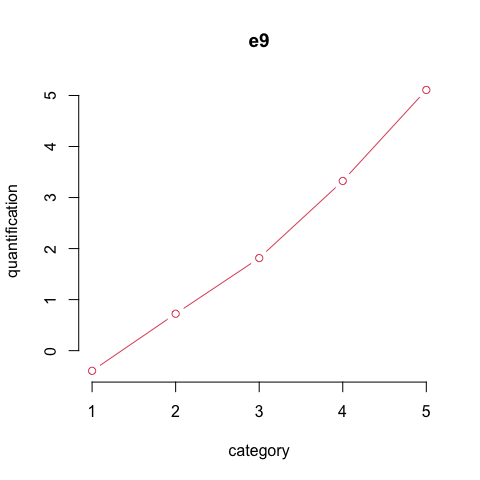#### Comparison of different penalty parameter values We can apply the function for different values of the penalty parameter. As we specify lambda as a (decreasing) vector, the output will result in a list of multivariate matrices. Note that optimization starts with the first component of lambda. Thus, if lambda is not in decreasing order, the vector will be sorted internally and so will be corresponding results. ehd_pca2 <- ordPCA(H, p = 2, lambda = c(5, 0.5, 0), maxit = 100, crit = 1e-7, qstart = NULL, Ks = apply(H, 2, max), constr = rep(TRUE, ncol(H)), CV = FALSE) ehd_pca2$pca: List of length corresponding to lambda, e.g. for $$\lambda=0$$:

summary(ehd_pca2$pca[]) #> Importance of components: #> PC1 PC2 PC3 PC4 PC5 PC6 PC7 #> Standard deviation 2.2881 2.0538 1.3062 1.20639 1.05816 0.96313 0.83058 #> Proportion of Variance 0.2618 0.2109 0.0853 0.07277 0.05598 0.04638 0.03449 #> Cumulative Proportion 0.2618 0.4727 0.5580 0.63073 0.68672 0.73310 0.76759 #> PC8 PC9 PC10 PC11 PC12 PC13 PC14 #> Standard deviation 0.82684 0.73470 0.70546 0.66612 0.65706 0.63456 0.57075 #> Proportion of Variance 0.03418 0.02699 0.02488 0.02219 0.02159 0.02013 0.01629 #> Cumulative Proportion 0.80178 0.82877 0.85365 0.87583 0.89742 0.91755 0.93384 #> PC15 PC16 PC17 PC18 PC19 PC20 #> Standard deviation 0.5458 0.52864 0.48645 0.44536 0.42629 0.35923 #> Proportion of Variance 0.0149 0.01397 0.01183 0.00992 0.00909 0.00645 #> Cumulative Proportion 0.9487 0.96271 0.97454 0.98446 0.99355 1.00000 ehd_pca2$qs: List of matrices (of dimension Ks x length(lambda)) with entries corresponding to the variables, e.g. for the second variable (e9):

ehd_pca2$qs[] #> [,1] [,2] [,3] #> [1,] -0.4046272 -0.3957913 -0.3795494 #> [2,] 0.7922099 0.7232103 0.9765504 #> [3,] 1.9948217 1.8145257 1.2336156 #> [4,] 3.2505365 3.3247656 3.0541415 #> [5,] 4.5318967 5.1081132 8.2666190 With an increasing penalty parameter, quantifications become increasingly linear: plot(ehd_pca2$qs[][,3], type = "b", xlab = "category", ylab = "quantification", col = 1,
ylim = range(ehd_pca2$qs[]), main = colnames(H), bty = "n") lines(ehd_pca2$qs[][,2], type = "b", col = 2, lty = 2, pch = 2, lwd=2)
lines(ehd_pca2$qs[][,1], type = "b", col = 3, lty = 3, pch = 3, lwd=2)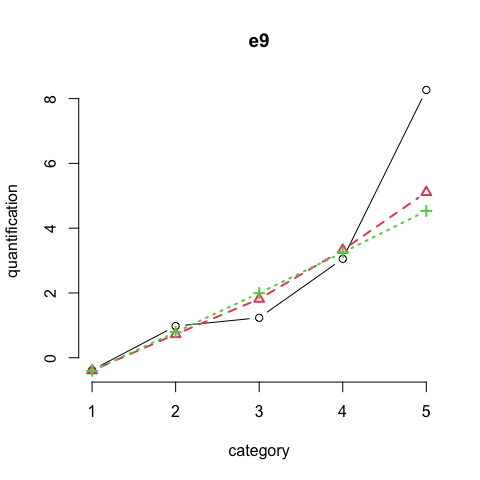Visualizing some variables: par(mar = c(4.1, 4.1, 3.1, 1.1)) for(j in c(1, 9, 12, 13, 15, 19)){ plot(ehd_pca2$qs[[j]][,3], type = "b", main = colnames(H)[j], xlab = "category",
ylab = "quantification", lwd = 2, bty = "n")
lines(ehd_pca2$qs[[j]][,2], type = "b", col = 2, lty = 2, pch = 2, lwd = 2) lines(ehd_pca2$qs[[j]][,1], type = "b", col = 3, lty = 3, pch = 3, lwd = 2)
}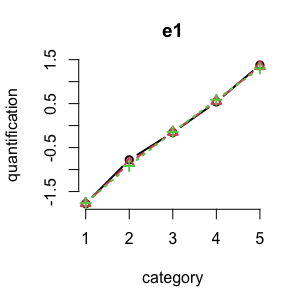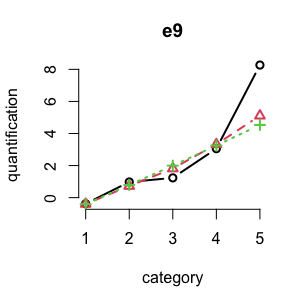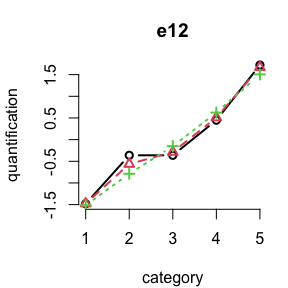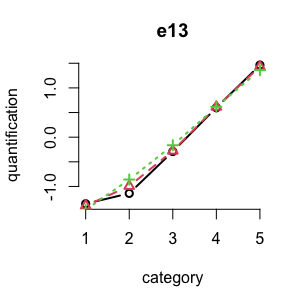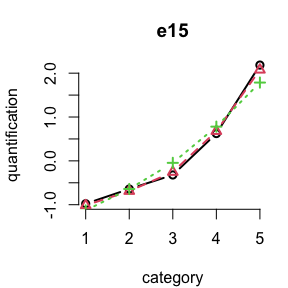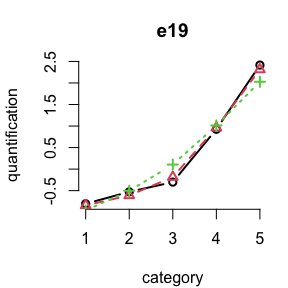#### Comparison of VAF for different penalties

We can also compare different amounts of penalization by means of variance accounted for (VAF) for performance evaluation by setting CV = TRUE and specifying the number of folds k. The resulting output is a matrix with columns corresponding to lambda and rows corresponding to the folds k. Use CVfit = TRUE to perform both k-fold cross validation and estimation (which, however, can be time-consuming if lambda has many elements).

ehd_pca3 <- ordPCA(H, p = 2, lambda = c(5, 0.5, 0.001), maxit = 100, crit = 1e-7,
qstart = NULL, Ks = apply(H, 2, max), constr = rep(TRUE, ncol(H)),
CV = TRUE, k = 5)

ehd_pca3$VAFtest #> [,1] [,2] [,3] #> [1,] 0.5057916 0.5137599 0.5167704 #> [2,] 0.5258273 0.5233661 0.5221925 #> [3,] 0.4026390 0.4059655 0.4049392 #> [4,] 0.4665078 0.4519429 0.4434176 #> [5,] 0.4992986 0.5014622 0.4970469 #### k-fold Cross Validation For selecting the right amount of penalization, however, k-fold cross validation should be performed over a fine grid of (many) sensible values $$\lambda$$. Due to time-consuming computation and undesireably high dimensions of outputs, we recommend to set the default CVfit = FALSE. By doing so, the function only stores VAF values for both the training set and the validation set. In addiction, the function provides the option of both non-monotone effects and incorporating constraints enforcing monotonicity, specified by the logical argument constr. For the ehd data the assumption of monotonic effects seems reasonable. lambda <- 10^seq(4, -4, by = -0.1) set.seed(456) ehd_CV_p2 <- ordPCA(H, p = 2, lambda = lambda, maxit = 100, crit = 1e-7, Ks = apply(H, 2, max), qstart = NULL, constr = rep(TRUE, ncol(H)), CV = TRUE, k = 5, CVfit = FALSE) lam_p2 <- (lambda)[which.max(apply(ehd_CV_p2$VAFtest,2,mean))]
ehd_CV_p2$VAFtest #> [,1] [,2] [,3] [,4] [,5] [,6] [,7] #> [1,] 0.4562018 0.4562027 0.4562039 0.4562054 0.4562072 0.4562095 0.4562124 #> [2,] 0.4763869 0.4763883 0.4763901 0.4763923 0.4763950 0.4763985 0.4764029 #> [3,] 0.4465576 0.4465585 0.4465597 0.4465611 0.4465630 0.4465653 0.4465682 #> [4,] 0.5237630 0.5237643 0.5237659 0.5237678 0.5237703 0.5237734 0.5237774 #> [5,] 0.4730329 0.4730340 0.4730354 0.4730371 0.4730393 0.4730420 0.4730454 #> [,8] [,9] [,10] [,11] [,12] [,13] [,14] #> [1,] 0.4562161 0.4562207 0.4562264 0.4562337 0.4562428 0.4562542 0.4562685 #> [2,] 0.4764084 0.4764153 0.4764240 0.4764350 0.4764487 0.4764660 0.4764876 #> [3,] 0.4465718 0.4465764 0.4465822 0.4465894 0.4465985 0.4466099 0.4466242 #> [4,] 0.5237823 0.5237885 0.5237963 0.5238061 0.5238185 0.5238339 0.5238533 #> [5,] 0.4730497 0.4730551 0.4730619 0.4730705 0.4730812 0.4730946 0.4731114 #> [,15] [,16] [,17] [,18] [,19] [,20] [,21] #> [1,] 0.4562864 0.4563091 0.4563372 0.4563723 0.4564158 0.4564698 0.4565365 #> [2,] 0.4765147 0.4765493 0.4765920 0.4766454 0.4767121 0.4767950 0.4768978 #> [3,] 0.4466421 0.4466648 0.4466929 0.4467278 0.4467712 0.4468250 0.4468914 #> [4,] 0.5238776 0.5239084 0.5239466 0.5239943 0.5240537 0.5241275 0.5242188 #> [5,] 0.4731325 0.4731593 0.4731923 0.4732335 0.4732847 0.4733481 0.4734263 #> [,22] [,23] [,24] [,25] [,26] [,27] [,28] #> [1,] 0.4566184 0.4567184 0.4568396 0.4569852 0.4571621 0.4573675 0.4576054 #> [2,] 0.4770250 0.4771814 0.4773728 0.4776054 0.4778919 0.4782304 0.4786310 #> [3,] 0.4469728 0.4470720 0.4471919 0.4473356 0.4475094 0.4477097 0.4479398 #> [4,] 0.5243314 0.5244695 0.5246378 0.5248412 0.5250893 0.5253807 0.5257221 #> [5,] 0.4735223 0.4736394 0.4737810 0.4739507 0.4741555 0.4743926 0.4746654 #> [,29] [,30] [,31] [,32] [,33] [,34] [,35] #> [1,] 0.4578763 0.4581851 0.4585184 0.4588735 0.4592497 0.4596216 0.4599897 #> [2,] 0.4790991 0.4796486 0.4802648 0.4809508 0.4817148 0.4825217 0.4833808 #> [3,] 0.4481987 0.4484894 0.4487958 0.4491184 0.4494360 0.4497446 0.4500211 #> [4,] 0.5261160 0.5265701 0.5270712 0.5276241 0.5282098 0.5288264 0.5294480 #> [5,] 0.4749732 0.4753184 0.4756858 0.4760691 0.4764617 0.4768387 0.4771945 #> [,36] [,37] [,38] [,39] [,40] [,41] [,42] #> [1,] 0.4603319 0.4606287 0.4608768 0.4610542 0.4611597 0.4611790 0.4611137 #> [2,] 0.4842490 0.4851322 0.4859840 0.4868130 0.4875745 0.4882822 0.4888944 #> [3,] 0.4502637 0.4504552 0.4506024 0.4507030 0.4507609 0.4507931 0.4508058 #> [4,] 0.5300704 0.5306708 0.5312268 0.5317346 0.5321736 0.5325299 0.5327917 #> [5,] 0.4775075 0.4777759 0.4779887 0.4781518 0.4782675 0.4783442 0.4783922 #> [,43] [,44] [,45] [,46] [,47] [,48] [,49] #> [1,] 0.4609601 0.4607229 0.4604067 0.4600177 0.4596415 0.4592546 0.4588131 #> [2,] 0.4894113 0.4898415 0.4901455 0.4904646 0.4907380 0.4908885 0.4909116 #> [3,] 0.4508160 0.4508725 0.4510101 0.4511376 0.4512577 0.4514542 0.4517094 #> [4,] 0.5329492 0.5329946 0.5329225 0.5327305 0.5324236 0.5321435 0.5317465 #> [5,] 0.4784219 0.4784416 0.4784532 0.4784642 0.4784765 0.4784777 0.4784661 #> [,50] [,51] [,52] [,53] [,54] [,55] [,56] #> [1,] 0.4583423 0.4578553 0.4574154 0.4570382 0.4566423 0.4562782 0.4560226 #> [2,] 0.4907850 0.4905980 0.4903708 0.4901828 0.4900356 0.4899373 0.4897991 #> [3,] 0.4519462 0.4521686 0.4523213 0.4524094 0.4524397 0.4524574 0.4524645 #> [4,] 0.5312765 0.5308215 0.5304965 0.5302086 0.5299833 0.5298190 0.5297227 #> [5,] 0.4784541 0.4784108 0.4783794 0.4783348 0.4782991 0.4782673 0.4782383 #> [,57] [,58] [,59] [,60] [,61] [,62] [,63] #> [1,] 0.4557980 0.4556046 0.4554319 0.4552787 0.4551440 0.4550337 0.4549337 #> [2,] 0.4896392 0.4894830 0.4893340 0.4891949 0.4890784 0.4889671 0.4888785 #> [3,] 0.4524575 0.4525033 0.4525448 0.4525758 0.4525989 0.4526176 0.4526313 #> [4,] 0.5296651 0.5296170 0.5295830 0.5295544 0.5295282 0.5294757 0.5294374 #> [5,] 0.4782119 0.4781878 0.4781700 0.4780690 0.4779855 0.4779200 0.4778577 #> [,64] [,65] [,66] [,67] [,68] [,69] [,70] #> [1,] 0.4548553 0.4547835 0.4547215 0.4546769 0.4546356 0.4546082 0.4545815 #> [2,] 0.4887941 0.4887314 0.4886711 0.4886161 0.4885806 0.4885462 0.4885286 #> [3,] 0.4526405 0.4526480 0.4526535 0.4526577 0.4526612 0.4526633 0.4526643 #> [4,] 0.5294109 0.5293872 0.5293719 0.5293572 0.5293440 0.5293374 0.5293307 #> [5,] 0.4778105 0.4777655 0.4777335 0.4777024 0.4776740 0.4776564 0.4776394 #> [,71] [,72] [,73] [,74] [,75] [,76] [,77] #> [1,] 0.4545676 0.4545423 0.4545307 0.4545193 0.4545085 0.4544987 0.4544899 #> [2,] 0.4885104 0.4884924 0.4884752 0.4884591 0.4884442 0.4884305 0.4884182 #> [3,] 0.4526651 0.4526661 0.4526668 0.4526672 0.4526673 0.4526670 0.4526666 #> [4,] 0.5293243 0.5293183 0.5293128 0.5293078 0.5293033 0.5292992 0.5292954 #> [5,] 0.4776310 0.4776224 0.4776138 0.4776054 0.4775974 0.4775899 0.4775829 #> [,78] [,79] [,80] [,81] #> [1,] 0.4544821 0.4544753 0.4544694 0.4544643 #> [2,] 0.4884070 0.4883971 0.4883882 0.4883804 #> [3,] 0.4526660 0.4526653 0.4526646 0.4526638 #> [4,] 0.5292921 0.5292890 0.5292863 0.5292838 #> [5,] 0.4775766 0.4775708 0.4775656 0.4775610 The optimal $$\lambda$$ can then be determined by the value maximizing the cross-validated VAF on the validation set, averaged over folds: plot(log10(lambda), apply(ehd_CV_p2$VAFtrain,2,mean), type = "l",
xlab = expression(log(lambda)), ylab = "proportion of variance explained",
main = "training data", cex.axis = 1.2, cex.lab = 1.2, cex.main = 1.4)
plot(log10(lambda), apply(ehd_CV_p2$VAFtest,2,mean), type = "l", xlab = expression(log(lambda)), ylab = "proportion of variance explained", main = "validation data", cex.axis = 1.2, cex.lab = 1.2, cex.main = 1.4) abline(v = log10(lambda)[which.max(apply(ehd_CV_p2$VAFtest,2,mean))])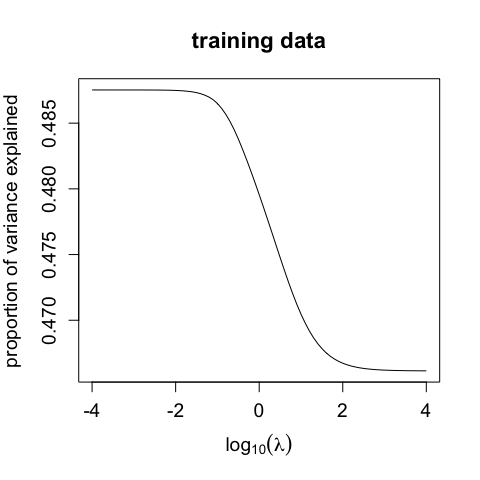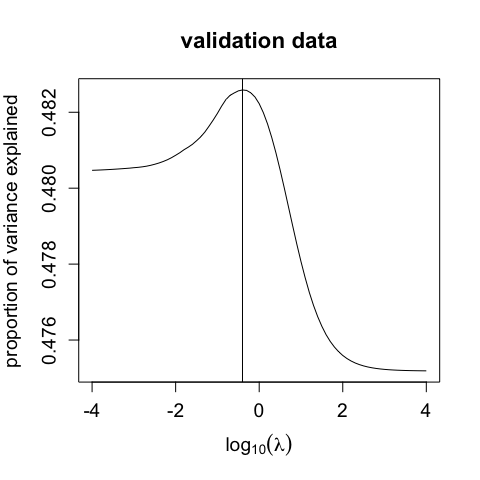#### Selecting the number of components

Note, that the choice of $$\lambda$$ relies on a fixed number of components p, which must be specified before $$\lambda$$ is selected. One strategy for selecting an appropriate number of components, is to use the elbow of the scree plot for standard linear PCA as an initial guess. To make sure that the choice is valid, we could then look at different scree plots when extracting different p’s in that area (here: p=1, p=2, p=3, p=4) and inserting the respective optimal $$\lambda$$ value:

# evaluate model with optimal lambda for p=2
ehd_pca_p2 <- ordPCA(H, p=2, lambda = lam_p2, Ks=apply(H,2,max), constr=rep(TRUE,ncol(H)))

# evaluate optimal lambda & model for p=1, p=3, p=4
set.seed(456)
ehd_CV_p1 <- ordPCA(H, p = 1, lambda=lambda, constr = rep(TRUE, ncol(H)), CV = TRUE, k = 5)
ehd_CV_p3 <- ordPCA(H, p = 3, lambda=lambda, constr = rep(TRUE, ncol(H)), CV = TRUE, k = 5)
ehd_CV_p4 <- ordPCA(H, p = 4, lambda=lambda, constr = rep(TRUE, ncol(H)), CV = TRUE, k = 5)

lam_p1 <- (lambda)[which.max(apply(ehd_CV_p1$VAFtest,2,mean))] lam_p3 <- (lambda)[which.max(apply(ehd_CV_p3$VAFtest,2,mean))]
lam_p4 <- (lambda)[which.max(apply(ehd_CV_p4$VAFtest,2,mean))] ehd_pca_p1 <- ordPCA(H, p=3, lambda=lam_p1, Ks=apply(H,2,max), constr=rep(TRUE,ncol(H))) ehd_pca_p3 <- ordPCA(H, p=3, lambda=lam_p1, Ks=apply(H,2,max), constr=rep(TRUE,ncol(H))) ehd_pca_p4 <- ordPCA(H, p=4, lambda=lam_p1, Ks=apply(H,2,max), constr=rep(TRUE,ncol(H))) #### Selecting the number of components Note, that the choice of $$\lambda$$ relies on a fixed number of components p, which must be specified before $$\lambda$$ is selected. One strategy for selecting an appropriate number of components, is to use the elbow of the scree plot for standard linear PCA as an initial guess. To make sure that the choice is valid, we could then look at different scree plots when extracting different p’s in that area (here: p=1, p=2, p=3, p=4) and inserting the respective optimal $$\lambda$$ value: # evaluate model with optimal lambda for p=2 ehd_pca_p2 <- ordPCA(H, p=2, lambda = lam_p2, Ks=apply(H,2,max), constr=rep(TRUE,ncol(H))) # evaluate optimal lambda & model for p=1, p=3, p=4 set.seed(456) ehd_CV_p1 <- ordPCA(H, p = 1, lambda=lambda, constr = rep(TRUE, ncol(H)), CV = TRUE, k = 5) ehd_CV_p3 <- ordPCA(H, p = 3, lambda=lambda, constr = rep(TRUE, ncol(H)), CV = TRUE, k = 5) ehd_CV_p4 <- ordPCA(H, p = 4, lambda=lambda, constr = rep(TRUE, ncol(H)), CV = TRUE, k = 5) lam_p1 <- (lambda)[which.max(apply(ehd_CV_p1$VAFtest,2,mean))]
lam_p3 <- (lambda)[which.max(apply(ehd_CV_p3$VAFtest,2,mean))] lam_p4 <- (lambda)[which.max(apply(ehd_CV_p4$VAFtest,2,mean))]

ehd_pca_p1 <- ordPCA(H, p=3, lambda=lam_p1, Ks=apply(H,2,max), constr=rep(TRUE,ncol(H)))
ehd_pca_p3 <- ordPCA(H, p=3, lambda=lam_p1, Ks=apply(H,2,max), constr=rep(TRUE,ncol(H)))
ehd_pca_p4 <- ordPCA(H, p=4, lambda=lam_p1, Ks=apply(H,2,max), constr=rep(TRUE,ncol(H)))

plot(ehd_pca_p1$pca$sdev[1:10]^2, bty="n",  xaxt="n", type="o", main=NULL, xlab="", pch=19,
ylab="Variances", ylim=range(c(ehd_pca_p1$pca$sdev^2, prcomp(H, scale=T)$sdev^2)), col=6) lines(1:10, ehd_pca_p2$pca$sdev[1:10]^2, col = 2, type = "o", pch = 19) lines(1:10, ehd_pca_p3$pca$sdev[1:10]^2, col = 3, type = "o", pch = 19) lines(1:10, ehd_pca_p4$pca$sdev[1:10]^2, col = 4, type = "o", pch = 19) lines(1:10, prcomp(H, scale = T)$sdev[1:10]^2, col = 1, type = "o", pch = 19)
legend(8, 5, legend=c("p=1","p=2","p=3","p=4","std"), col=c(6,2:4,1), lty=1, bty="n")
axis(1, at = 1:10, labels = 1:10)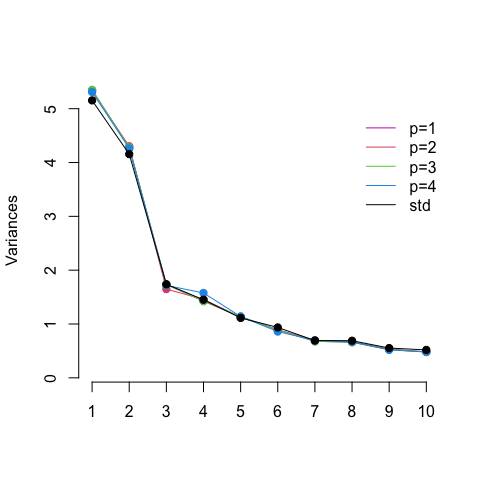As can be seen for different p values, for the ehd data, the first two components are by far the most relevant. This can also be confirmed when viewing the scree plot for standard linear PCA.

d4b1b5e8bdb2525c677c2bebababe2e56804a737

plot(ehd_pca_p1$$pca$$sdev[1:10]^2, bty=“n”, xaxt=“n”, type=“o”, main=NULL, xlab="“, pch=19, ylab=”Variances“, ylim=range(c(ehd_pca_p1$$pca$$sdev^2, prcomp(H, scale=T)$$sdev^2)), col=6) lines(1:10, ehd_pca_p2$$pca$$sdev[1:10]^2, col = 2, type = "o", pch = 19) lines(1:10, ehd_pca_p3$$pca$$sdev[1:10]^2, col = 3, type = "o", pch = 19) lines(1:10, ehd_pca_p4$$pca$$sdev[1:10]^2, col = 4, type = "o", pch = 19) lines(1:10, prcomp(H, scale = T)$$sdev[1:10]^2, col = 1, type =”o“, pch = 19) legend(8, 5, legend=c(”p=1“,”p=2“,”p=3“,”p=4“,”std“), col=c(6,2:4,1), lty=1, bty=”n") axis(1, at = 1:10, labels = 1:10)


As can be seen for different p values, for the ehd data, the first two components are by far the most relevant. This can also be confirmed when viewing the scree plot for standard linear PCA.

### ICF data example
#### Monotonicity assumptions
The International Classification of Functioning, Disability and Health (ICF) core set data for chronic widespread pain (CWP) available in the ordPens package consists of 420 observations of 67 ordinally scaled variables, each one associated with one of the following four types: 'body functions', 'body structures', 'activities and participation', and 'environmental factors'.
The latter are measured on a nine-point Likert scale ranging from −4 ‘complete barrier’ to +4 ‘complete facilitator’. All remaining factors are evaluated on a five-point Likert scale ranging from 0 ‘no problem’ to 4 ‘complete problem’.
For a detailed view see Cieza et al. (2004) and Gertheiss et al. (2011) or type ?ICFCoreSetCWP.

Again, we need to make sure that the ordinal design matrix is coded adequately:

r
data(ICFCoreSetCWP)
H <- ICFCoreSetCWP[, 1:67] + matrix(c(rep(1,50), rep(5,16), 1),
nrow(ICFCoreSetCWP), 67, byrow = TRUE)
#>   b1602 b122 b126 b130 b134 b140 b147 b152 b164 b180 b260 b265 b270 b280 b430
#> 1     1    2    3    2    2    1    1    3    1    1    1    1    1    2    1
#> 2     4    3    3    4    4    3    4    4    4    2    3    3    3    3    1
#> 3     1    2    3    2    2    1    2    3    1    1    1    1    1    2    1
#> 4     1    1    1    3    2    1    1    1    1    1    1    1    1    4    1
#> 5     1    1    1    1    1    1    1    1    1    1    1    1    1    1    1
#> 7     1    2    1    3    4    4    3    4    3    3    1    1    4    4    1
#>   b455 b640 b710 b730 b735 b740 b760 b780 d160 d175 d220 d230 d240 d410 d415
#> 1    2    1    1    2    1    1    1    2    1    1    2    1    2    1    1
#> 2    4    1    3    4    4    4    4    4    4    3    4    4    4    4    4
#> 3    2    1    1    2    1    2    1    2    1    1    1    2    2    1    1
#> 4    4    1    1    1    1    4    1    2    1    1    1    2    1    1    3
#> 5    1    2    1    1    1    1    1    1    1    1    1    1    1    1    1
#> 7    4    3    1    4    4    4    1    3    3    3    4    3    1    4    4
#>   d430 d450 d455 d470 d475 d510 d540 d570 d620 d640 d650 d660 d720 d760 d770
#> 1    2    1    3    1    2    1    1    1    2    1    1    1    1    1    1
#> 2    4    4    4    4    1    3    3    4    4    4    4    4    4    4    1
#> 3    2    1    3    1    2    1    1    1    1    1    1    1    1    1    1
#> 4    3    4    3    1    1    1    1    1    1    2    1    1    1    1    1
#> 5    1    1    1    1    1    1    1    1    1    1    1    1    1    1    1
#> 7    4    2    4    2    4    2    2    2    2    4    3    1    2    1    4
#>   d845 d850 d855 d910 d920 e1101 e310 e325 e355 e410 e420 e425 e430 e450 e455
#> 1    1    1    1    1    2     5    5    5    9    5    5    5    5    9    5
#> 2    1    1    3    3    3     7    8    7    8    8    8    8    8    8    8
#> 3    1    1    1    1    2     5    5    5    9    5    5    5    5    9    5
#> 4    1    1    1    1    1     5    7    6    7    5    4    4    5    7    7
#> 5    1    1    1    1    1     5    6    5    6    5    5    5    5    6    5
#> 7    3    3    1    1    3     8    8    8    8    8    7    6    4    7    7
#>   e460 e465 e570 e575 e580 e590 s770
#> 1    5    5    8    8    9    8    1
#> 2    7    7    7    7    7    7    3
#> 3    5    5    8    8    9    5    1
#> 4    4    5    5    7    7    6    2
#> 5    5    5    5    5    5    5    1
#> 7    3    3    6    6    6    6    2
xnames <- colnames(H)

To this point, we assumed the quantifications to increase or decrease monotonically, as in the previous example, the affected variables of the ehd data are supposed to have consistent, negative association with depressive mood.

Monotonicity, however, is not always a reasonable assumption. For the environmental factors from the ICF, in particular, also non-monotone transformations could make sense, while for the other variables monotonicity seems reasonable.

We can simply specify for which variables a monotonicity constraint is to be applied by the logical argument constr. Indeed, non-monotonicity is detected for the environmental factors (prefix ‘e’):

icf_pca1 <- ordPCA(H, p = 2, lambda = c(5, 0.5, 0.001), maxit = 100, crit = 1e-7, qstart = NULL,
Ks = c(rep(5,50), rep(9,16), 5),
constr = c(rep(TRUE,50), rep(FALSE,16), TRUE),
CV = FALSE, k = 5, CVfit = FALSE)

icf_pca1C <- ordPCA(H, p = 2, lambda = c(5, 0.5, 0.001), maxit = 100, crit = 1e-7, qstart = NULL,
Ks = c(rep(5,50), rep(9,16), 5), constr = rep(TRUE, ncol(H)),
CV = FALSE, k = 5, CVfit = FALSE)

plot(icf_pca1$qs[][,3], type = "b", xlab = "category", ylab = "quantification", col = 1, ylim = range(icf_pca1$qs[]), main = xnames, bty = "n", xaxt = "n")
lines(icf_pca1$qs[][,2], type = "b", col = 2, lty = 2, pch = 2, lwd=2) lines(icf_pca1$qs[][,1], type = "b", col = 3, lty = 3, pch = 3, lwd=2)
axis(1, at = 1:length(icf_pca1$qs[][,1]), labels = -4:4) plot(icf_pca1C$qs[][,3], type = "b", xlab = "category", ylab = "quantification", col = 1,
ylim = range(icf_pca1C$qs[]), main = xnames, bty = "n", xaxt = "n") lines(icf_pca1C$qs[][,2], type = "b", col = 2, lty = 2, pch = 2, lwd=2)
lines(icf_pca1C$qs[][,1], type = "b", col = 3, lty = 3, pch = 3, lwd=2) axis(1, at = 1:length(icf_pca1C$qs[][,1]), labels = -4:4)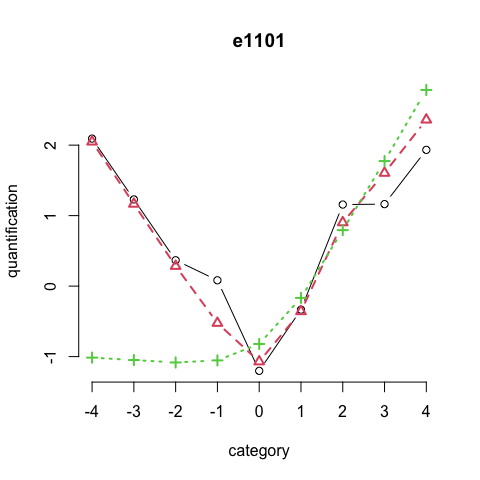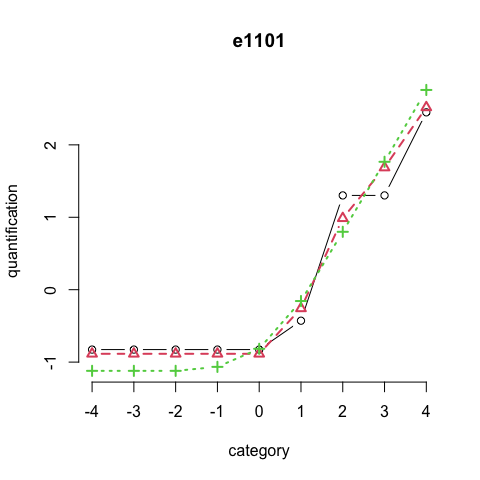It can be seen, that monotonicity constraints (right) on the environmental factors clearly distort valuable information contained in the negative categories/barriers.

Thus, in the preferred model, monotonicity constraints are only applied to variables corresponding to ‘body functions’, ‘body structures’, ‘activities and participation’.

Visualizing some variables:

wx <- which(xnames=="b265"|xnames=="d450"|xnames=="d455"|xnames=="e1101"|xnames=="e460"
|xnames=="e325")
xmain <- c()
xmain[wx] <- list("Touch function",
"Walking",
"Moving around",
"Drugs",
"Societal attitudes",
paste(c("Acquaintances,colleagues,","peers,community members")))

par(mar = c(4.1, 4.1, 3.1, 1.1))
for (j in wx){
plot(icf_pca1$qs[[j]][,3], type = "b", main = xmain[j], xlab = "category", bty = "n", ylim = range(icf_pca1$qs[[j]]), ylab = "quantification", xaxt = "n", cex.main= 1)
lines(icf_pca1$qs[[j]][,2], type = "b", col = 2, lty = 2, pch = 2, lwd=2) lines(icf_pca1$qs[[j]][,1], type = "b", col = 3, lty = 3, pch = 3, lwd=2)
axis(1, at = 1:length(icf_pca1$qs[[j]][,1]), labels = ((1:length(icf_pca1$qs[[j]][,1])) - c(rep(1,50), rep(5,16), 1)[j]))
}`